Question 1

A block of mass m is in contact with the cart C as shown in figure -
The coefficient of static friction between the block and the cart is µ. The acceleration α of the cart that will prevent the block from falling satisfies -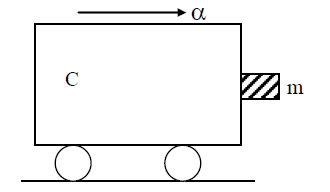A : α > mg/ µ
B : α > g/ µm
C : α >= g/ µ
D : α < g/ µ

•
.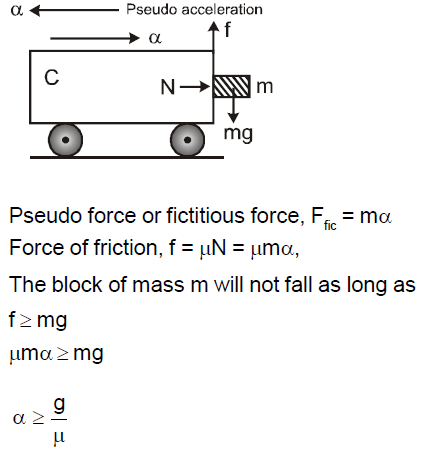•   Question 2

Themass of a 73Li nucleus is 0.042u less than the sum of the masses of all its nucleons. The binding energy per nucleon of its nucleus is nearly

A : 46 MeV
B : 5.6 MeV
C : 3.9 MeV
D : 23 MeV

•
.

Solution :
BE/nucleon = 0.042 * 931 / 7
= 5.6 MeV

•   Question 3

A circular disk of moment of inertia It is rotating in a horizontal plane, about its symmetry axis, with a constant angular speed ωr. Another disk of moment of inertia Ib is dropped coaxially onto the rotating disk. Initially the second disk has zero angular speed. Eventually both the disks rotate with a constant angular speed ωf. The energy lost by the initially rotating disc to friction is

A : 1/2 Ib2 / (It + Ibi2
B : 1/2 It2 / (It + Ibi2
C : (Ib - It) / (It + Ibi2
D : 1/2 IbIt / (It + Ibi2

•
.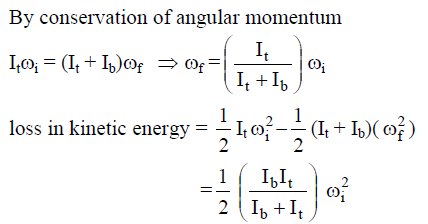•   Question 4

Which one of the following statement is false?

A : Pure Si doped with trivalent impurities gives a p-type semiconductor.
B : Majority carriers in a n-type semiconductor are holes.
C : Minority carriers in a p-type semiconductor are electrons.
D : The resistance of intrinisic semiconductor decreases with increase of temperature.

•
.

Solution :
In a n-type semiconductors, electrons are majority carriers and holes are minority carriers.

•   Question 5

The displacement of a particle along the x axis is given by x = a sin2 ωt. Themotion of the particle corresponds to

A : simple harmonic motion of frequency ω/π
B : simple harmonic motion of frequency 3ω/2π
C : non simple harmonic motion
D : simple harmonic motion of frequency ω/2π

•
.

Solution :
x = asin2ωt
=a/2 (1 - cos2ωt)

•   Question 6

The displacement of a particle along the x axis is given by x = a sin2 ωt. Themotion of the particle corresponds to

A : simple harmonic motion of frequency ω/π
B : simple harmonic motion of frequency 3ω/2π
C : non simple harmonic motion
D : simple harmonic motion of frequency ω/2π

•
.

Solution :
x = asin2ωt
=a/2 (1 - cos2ωt)

•   Question 7

The radii of circular orbits of two satellites A and B of the earth, are 4R and R, respectively. If the speed of satellite A is 3V, then the speed of satellite B will be

A : 3V/4
B : 6V
C : 12V
D : 3V/2

•
.

Solution :
Speed of satellite V = √(GM/r)
VB / VA = √(rA / rB) = √(4R / R) =2
VB = (3V)(2) =6V

•   Question 8

A beam of cathode rays is subjected to crossed electric (E) andmagnetic fields (B). The fields are adjusted such that the beam is not deflected. The specific charge of the cathode rays is given by
Where V is the potential difference between cathode and anode

A : B2 /2VE2
B : 2VB2 / E2
C : 2VE2 / B2
D : E2 / 2VB2

•
.

Solution :
qvB =qE => v=E/B
but 1/2 mv2 =qV
so q/m = v2/2V
= E2/2VB2

•   Question 9

A ball is dropped from a high platform at t = 0 starting from rest. After 6 seconds another ball is thrown downwards from the same platform with a speed v. The two balls meet at t = 18 s. What is the value of v? (Take g = 10 m/s2)

A : 75 m/s
B : 55 m/s
C : 40 m/s
D : 60 m/s

•
.

Solution :
Let two balls meet at depth h from platform
h = 1/2 g(18)*(18)
=v(12)+ 1/2 (12)*(12)
v = 75 ms-1

•   Question 10

A ray of light travelling in a transparent medium of refractive index µ, falls on a surface separating themedium from air at an angle of incidence of 45°C. For which of the following value of µ the ray can undergo total internal reflection?

A : 1.33
B : 1.40
C : 1.50
D : 1.25

•
.

Solution :
For TOTAL INTERNAL REFLECTION
45 >= ΘC
sin 45 >= sinΘC
1/√2 >= 1/µ
µ >= √2

•   Question 11

The period of oscillation of amass m suspended from a spring of negligiblemass is T. If along with it another mass M is also suspended the period of oscillation will now be

A : T
B : T / √2
C : 2T
D : √2 T

•
.

Solution :
T = 2π √ (M/k)
T' = 2π √ (2M/k)
=√2T

•   Question 12

A cylindrical metallic rod in thermal contact with two reservoirs of heat at its two ends conducts an amount of heat Q in time t. The metallic rod is melted and the material is formed into a rod of half the radius of the original rod.What is the amount of heat conducted by the new rod, when placed in thermal contact with the two reservoirs in time t?

A : Q/4
B : Q/16
C : 2Q
D : Q/2

•
.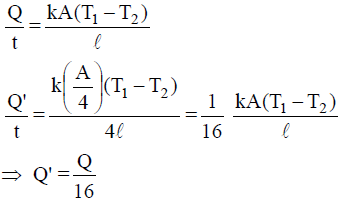•   Question 13

A ball moving with velocity 2 m/s collides head on with another stationary ball of double the mass. If the coefficient of restitution is 0.5, then their velocities (in m/s) after collision will be

A : 0, 1
B : 1, 1
C : 1, 0.5
D : 0, 2

•
.

Solution :
By conservation of linear momentum : 2m = mv1 + 2mv2
v1 + 2v2 = 2
by definition of e
e =1/2 = (v2 - v1) /(2-0)
v2 - v1 = 1
v1 = 0 and v2 = 1ms-1

•   Question 14

A transverse wave is represented by y =Asin(ωt - kx). For what value of the wavelength is the wave velocity equal to the maximum particle velocity?

A : πA/2
B : πA
C : 2πA
D : A

•
.

Solution :
Wave velocity = nλ = ωA λ = ωA /n
ωA / (ω/2π)
2πA

•   Question 15

A particle has initial velocity 3 î + 4 ĵ and has acceleration 0.4 î + 0.3 ĵ . Its speed after 10 s is

A : 7 units
B : 7√2 units
C : 8.5 units
D : 10 units

•
.

Solution :
v = u +at
(3 î + 4 ĵ) + (0.4 î + 0.3 ĵ)(10)
7 î + 7 ĵ
So speed = |v| = 7√2 ms-1

•   Question 16

An engine pumps water through a hose pipe.Water passes through the pipe and leaves it with a velocity of 2 m/s. The mass per unit length of water in the pipe is 100 kg/m. What is the power of the engine?

A : 400W
B : 200W
C : 100W
D : 800W

•
.

Solution :
Here,
Mass per unit length of water, µ = 100 kg/m
Velocity of water, v = 2m/s Power of engine, P = µv3
= (100 kg/m)(2m/s)3
= 800W

•   Question 17

A thin ring of radius Rmeter has charge q coulomb uniformly spread on it. The ring rotates about its axis with a constant frequency of f revolutions/s. The value of magnetic induction in Wb/m2 at the centre of the ring is

A : µ0qf / 2πR
B : µ0q / 2πfR
C : µ0q / 2fR
D : µ0qf / 2R

•
.

Solution :
Current produced due to circular motion of charge q is
I = qf
Magnetic field induction at the centre of the ring of radius R is
B = µ02πI / 4πR
0I / 2R
0qf / 2R

•   Question 18

Which one of the following bonds produces a solid that reflects light in the visible region and whose electrical conductivity decreases with temperature and has high melting point?

A : metallic bonding
B : van derWall?s bonding
C : ionic bonding
D : covalent bonding

•
.

•   Question 19

A particle moves a distance x in time t according to equation x = (t + 5)?-1. The acceleration of particle is proportional to

A : (velocity)3/2
B : (velocity)2
C : (velocity)-2
D : (velocity)2/3

•
.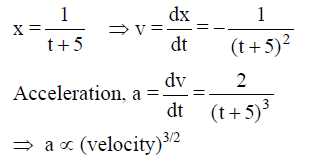•   Question 20

A conducting circular loop is placed in a uniform magnetic field, B = 0.025 T with its plane perpendicular to the loop. The radius of the loop is made to shrink at a constant rate of 1 mm s?-1. The induced emf when the radius is 2 cm, is

A : 2π µ V
B : π µ V
C : π/2 µ V
D : 2µ V

•
.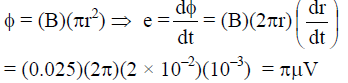•   Question 21

The activity of a radioactive sample is measured as N0 counts per minute at t = 0 and N0/e counts per minute at t = 5 minutes. The time (in minutes) at which the activity reduces to half its value is

A : loge 2/5
B : 5/(loge2)
C : 5log102
D : 5loge2

•
.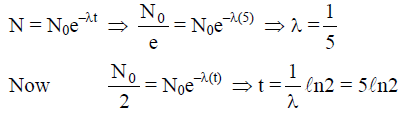•   Question 22

Two particles which are initially at rest,move towards each other under the action of their internal attraction. If their speeds are v and 2v at any instant, then the speed of centre of mass of the system will be

A : 2v
B : 0
C : 1.5v
D : v

•
.

Solution :
As no external force is acting on the system, the centre of mass must be at rest i.e. vCM = 0.

•   Question 23

A particle of mass M is situated at the center of a spherical shell of same mass and radius a. The gravitational potential at a point situated at a/2 distance from the centre, will be

A : -3GM/a
B : -2GM/a
C : -GM/a
D : -4GM/a

•
.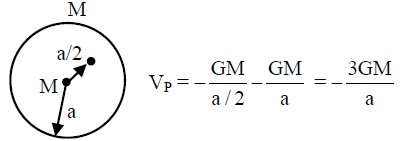•   Question 24

The device that act as a complete electronic circuit is

A : junction diode
B : integrated circuit
C : junction transistor
D : zener diode

•
.

•   Question 25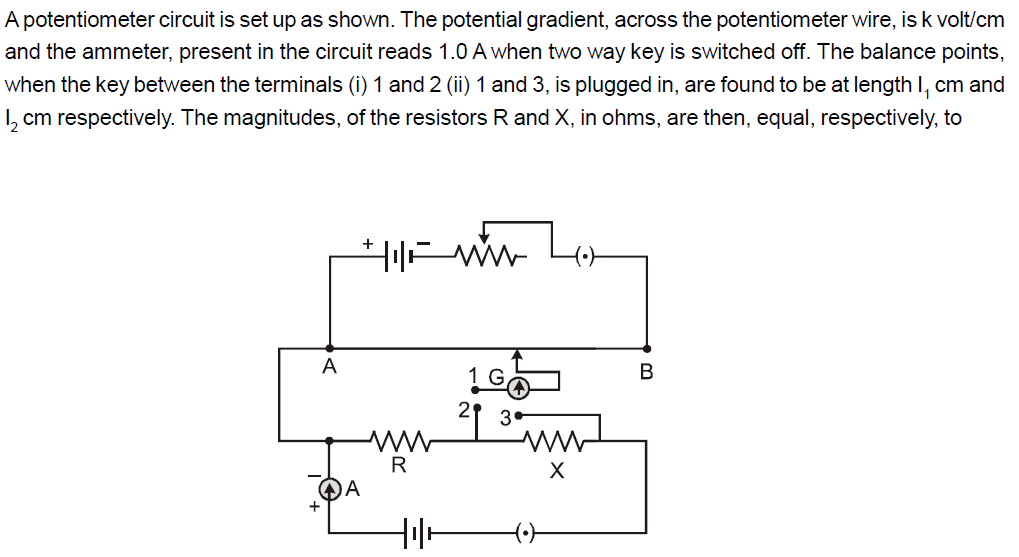A : k(l2 - l1) and kl2
B : kl1 and k(l2 - l1)
C : k(l2 - l1) and kl1
D : kl1 and kl2

•
.

Solution :
R = kl1 and R + X = kl2

•   Question 26

A tuning fork of frequency 512 Hz makes 4 beats per second with the vibrating string of a piano. The beat frequency decreases to 2 beats per sec when the tension in the piano string is slightly increased. The frequency of the piano string before increasing the tension was

A : 510 Hz
B : 514 Hz
C : 516 Hz
D : 508 Hz

•
.

Solution :
The frequency of the piano string may be 508 or 516 Hz.
As frequency ∝ √Tension so answer will be 508 Hz.

•   Question 27

Six vectors, ã through f̃ have the magnitudes and directions indicated in the figure.Which of the following statements is true?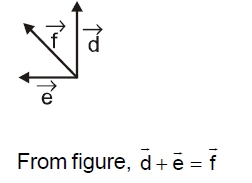A : b̃ + c̃ = f̃
B : d̃ + ̃ = f̃
C : d̃ + ẽ = f̃
D : b̃ + ẽ = f̃

•
.•   Question 28

A galvanometer has a coil of resistance 100 ohm and gives a full scale deflection for 30mA current. If it is to work as a voltmeter of 30 volt range, the resistance required to be added will be

A : 900 Ω
B : 1800 Ω
C : 500 Ω
D : 1000 Ω

•
.

Solution :
Let required resistance be R then (R + Rg)Ig = V
(R + 100) (30 � 10-3)
= 30
R = 900 Ω

•   Question 29

Which of the following statement is false for the properties of electromagnetic waves ?

A : both electric and magnetic field vectors attain the maxima and minima at the same place and same time
B : The energy in electromagnetic wave is divided equally between electric and magnetic vectors
C : Both electric and magnetic field vectors are parallel to each other and perpendicular to the direction of propagation of wave
D : These waves do not require any material medium for propagation

•
.

•   Question 30

The energy of a hydrogen atom in the ground state is - 13.6 eV. The energy of He+ ion in the first excited state will be

A : - 13.6 eV
B : - 27.2 eV
C : - 54.4 eV
D : - 6.8 eV

•
.

Solution :
En = -13.6 (Z2 / n2)
Z2 = 4
n2 = 4

•   Question 31

The dimension of 1/2 ε0 E2 , where ε0 is permittivity of free space and E is electric field, is

A : ML2T-2
B : ML-1-2
C : ML2T-1
D : MLT-1

•
.

Solution :
Energy density of an electric field E is
uE1/2 ε0 E2
Energy/Volume= (ML2T-2)/L3

•   Question 32

In producing chlorine by electrolysis 100 kW power at 125 V is being consumed. How much chlorine per minute is liberated (E.C.E. of chlorine is 0.367 * 10?6 kg/C)

A : 1.76 * 10?-3kg
B : 9.67 * 10?-3kg
C : 17.61 * 10?-3kg
D : 3.67 * 10?-3kg

•
.

Solution :
m = ZIt
Z(P/V)t
(0.367 * 10-6)*(100* 1000/125)*(60)

•   Question 33

Aman of 50 kgmass is standing in a gravity free space at a height of 10m above the floor. He throws a stone of 0.5 kg mass downwards with a speed 2 m/s. When the stone reaches the floor, the distance of the man above the floor will be

A : 9.9m
B : 10.1m
C : 10m
D : 20m

•
.

Solution :
Since the man is in gravity free space, force on man + stone system is zero Therefore centre ofmass of the system remains at rest. Let theman goes xmabovewhen the stone reaches the floor, then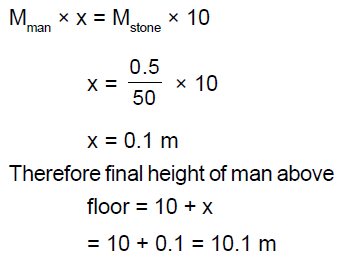•   Question 34

An alpha nucleus of energy 1/2 mu2 bombards a heavy nuclear target of charge Ze. Then the distance of closest approach for the alpha nucleus will be proportional to

A : 1/Ze
B : u2
C : 1/m
D : 1/u4

•
.

Solution :
1/2 mv2
= (Ze)(2e)/4πεdmin
then dmin ∝ 1/m

•   Question 35

A lens having focal length f and aperture of diameter d forms an image of intensity I.Aperture of diameter d/2 in central region of lens is covered by a block paper. Focal length of lens and intensity of image now will be respectively

A : f and I/4
B : 3f/4 and I/2
C : f and 3I/4
D : f/2 and I/2

•
.

Solution :
f' = f & Intensity ∝ Area
so I' = I - I/4 = 3I/4

•   Question 36

If ΔU and ΔW represent the increase in internal energy and work done by the system respectively in a thermodynamical process, which of the following is true?

A : ΔU = -ΔW, in a adiabatic process
B : ΔU = ΔW, in a isothermal process
C : ΔU = ΔW, in a adiabatic process
D : ΔU = -ΔW, in a isothermal process

•
.

Solution :
ΔQ = ΔU + ΔW In adiabatic process ΔQ = 0

•   Question 37

The total radiant energy per unit area, normal to the direction of incidence, received at a distance R from the center of a star of radius r, whose outer surface radiates as a black body at a temperature TK is given by (where σ is Stefan?s constant)

A : σr2T4 / R2
B : σr2T4 / 4 πr2
C : σr4T4 / r4
D : 4πσr2T4 / R2

•
.

Solution :
According to the stefan Boltzmann law, the power radiated by the star whose outer surface radiates as a black body at temperature T K is given by P = 4 πσr2T4
Total radiant energy per unit area
find
P/4πr2

•   Question 38

In the given circuit the reading of voltmeter V1 and V2 are 300 volts each. The reading of the voltmeter V3 and ammeterAare respectively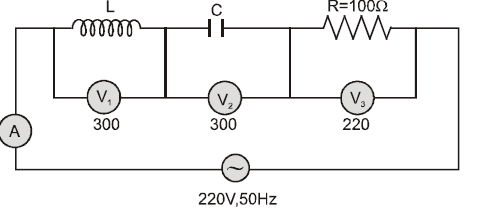A : 150 V, 2.2 A
B : 220 V, 2.2 A
C : 220 V, 2.0 A
D : 100 V, 2.0 A

•
.

Solution :
V3 = 220 volt, I = 220/100 = 2.2A

•   Question 39

A220 volt input is supplied to a transformer. The output circuit draws a current of 2.0 ampere at 440 volts. If the efficiency of the transformer is 80% the current drawn by the primary windings of the transformer is

A : 3.6 ampere
B : 2.8 ampere
C : 2.5 ampere
D : 5.0 ampere

•
.

Solution :
IP = VSIS/ VP*n

•   Question 40

A source S1 is producing 1015 photons per second of wavelength 5000Å. Another sorce S2 is producing 1.02 * 1015 photons per second of wavelength 5100 Å. Then (power of S2)/(power of S1) is equal to

A : 1.00
B : 1.02
C : 1.04
D : 0.98

•
.

Solution :
Power of S2 / Power of S1
= (n2(hc/λ2))/(n1(hc/λ1))

•   Question 41

A common emitter amplifier has a voltage gain of 50, an input impedance of 100 ohm and an output impedance of 200 ohm. The power gain of the amplifier is

A : 500
B : 1000
C : 1250
D : 50

•
.

Solution :
Voltage gain = Β(Rout / Rin )
Power gain = Β(Voltage gain)
25*50=1250

•   Question 42

A vibration magnetometer placed in magnetic meridian has a small bar magnet. The magnet executes oscillations with a time period of 2 sec in earth?s horizontal magnetic field of 24 microtesla. When a horizontal field of 18 microtesla is produced opposite to the earth?s field by placing a current carrying wire, the new time period of magnet will be

A : 1s
B : 2s
C : 3s
D : 4s

•
.

Solution :
The time period T of oscillation of a magnet is given by
T = 2π√(I/MB)

•   Question 43

Two positive ions, each carrying a charge q, are separated by a distance d. If F is the force of repulsion between the ions, the number of electrons missing from each ion will be (e being the charge on an electron)

A : 4πε0Fd2 / e2
B : √(4πε0Fe2 / d2)
C : √4πε0Fd2 / e2
D : 4πε0Fd2 / q2

•
.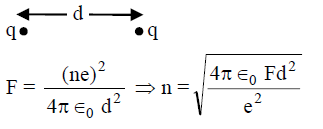•   Question 44

The potential difference thatmust be applied to stop the fastest photoelectrons emitted by a nickel surface, having work function 5.01 eV, when ultraviolet light of 200 nm falls on it, must be

A : 2.4V
B : -1.2V
C : -2.4V
D : 1.2V

•
.

Solution :
hv = Φ0 + eV0
where hv = 12400/2000 = 6.2eV
V0 =6.2 -5.01 = 1.20(approx)

•   Question 45

A square surface of side L meter in the plane of the paper is placed in a uniform electric field E (volt/m) acting along the same plane at an angle Θ with the horizontal side of the square as shown in figure. The electric flux linked to the surface in units of volt * meter is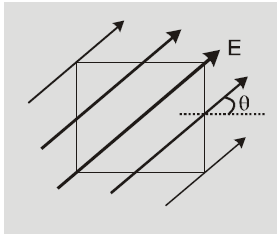A : EL2
B : EL2cosΘ
C : EL2sinΘ
D : 0

•
.

Solution :
Flux passing through area A
Φ = E.Acosθ
Φ = Ẽ . Ã = 0
lines are parallel to the surfaces

•   Question 46

A series combination of n1 capacitors, each of value C1 is charged by a source of potential difference 4V. When another parallel combination of n2 capacitors, each of value C2, is charged by a source of potential difference V, it has the same (total) energy stored in it, as the first combination has. The value of C2, in terms of C1, is then

A : 2C1/n1n2
B : 16n2C1/n1
C : 2n2C1/n1
D : 16C1/n1n2

•
.

Solution :
1/2 *(C1 /n1)*(4V)2
=1/2 * C2n2
C2 = 16C1/n1n2

•   Question 47

Electromagnets are made of soft iron because soft iron has

A : low retentivity and high coercive force
B : high retentivity and high coercive force
C : low retentivity and low coercive force
D : high retentivity and low coercive force

•
.

Solution :
Electromagnetics are made of soft iron because soft iron has low retentivity and low coercive force or low coercivity. Soft iron is a soft magnetic material

•   Question 48

A square current carrying loop is suspended in a uniform magnetic field acting in the plane of the loop. If the force on one arm of the loop is F̃ , the net force on the remaining three arms of the loop is

A : 3F̃
B : -F̃
C : -3F̃
D :

•
.

Solution :
Net force on loop is zero
force F acting on one side of loop
force ?F acting on remaining side of loop.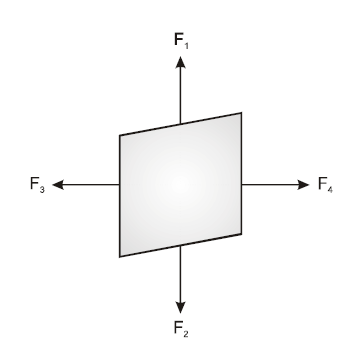•   Question 49

Consider the following two statements.
(A) Kirchhoff?s junction law follows from the conservation of charge
(B) Kirchhoff?s loop law follows from the conservation of energy
Which of the following is correct?

A : Both (A) and (B) are wrong
B : (A) is correct and (B) is wrong
C : (A) is wrong and (B) is correct
D : Both (A) and (B) are correct

•
.

Solution :
Kirchoff's junction law or Kirchhoff's first law is based on the conservation of charge. Kirchhoff's loop law or Kirchhoff's second law is based on the conservation of energy. Hence both statements (A) and (B) are correct

•   Question 50

To get an output Y = 1 from the circuit shown below the input must be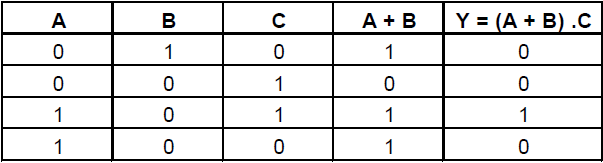A : A=0 B=1 C=0
B : A=0 B=0 C=1
C : A=1 B=0 C=1
D : A=1 B=0 C=0

•
.•   Question 51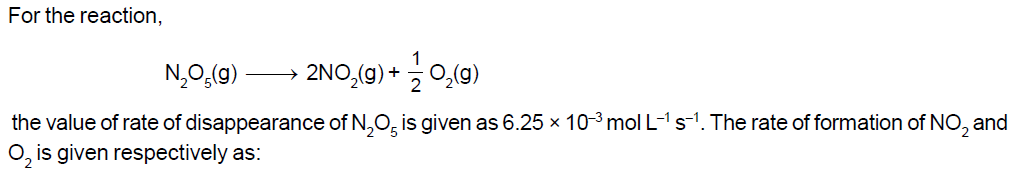A : 6.25 * 10-3 mol L-1 s-1 and 6.25 * 10?-3 mol L?-1s?-1
B : 1.25 * 10-2 mol L-1 s-1 and 3.125 * 10?-3 mol L?-1s?-1
C : 6.25 * 10-3 mol L-1 s-1 and 3.125 * 10?-3 mol L?-1s?-1
D : 1.25 * 10-2 mol L-1 s-1 and 6.25 * 10?-3 mol L?-1s?-1

•
.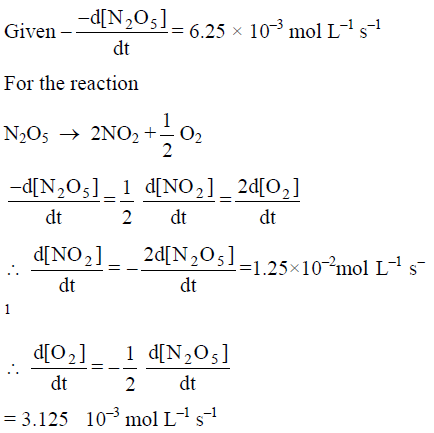•   Question 52

Liquid hydrocarbons can be converted to a mixture of gaseous hydrocarbons by:

A : Oxidation
B : Cracking
C : Distillation under reduced pressure
D : Hydrolysis

•
.

Solution :
Lower hydrocarbons exist in gaseous state while higher ones are in liquid state or solid state. On cracking or pyrolysis, the hydrocarbon with higher molecular mass gives a mixture of hydrocarbons having lower molecular mass. Hence, we can say that by cracking a liquid hydrocarbon can be converted into a mixture of gaseous hydrocarbons.

•   Question 53

In which of the following pairs of molecules/ions, the central atoms have sp2 hybridization ?

A : NO2- and NH3
B : BF3 and NO2-
C : NH2- and H2O
D : BF3 and NH2-

•
.

Solution :
NO2- has ? 2σ + 1lp = 3, i.e., sp2 hybridisation
BF3 has 2σ + 1lp = 3, i.e., sp2 hybridisation

•   Question 54

Which one of the following does not exhibit the phenomenon of mutarotation?

A : (+) Sucrose
B : (+) Lactose
C : (+) Maltose
D : (?) Fructose

•
.

Solution :
Reducing sugars that exist in hemiacetal and hemiketal forms, undergo mutarotation in aqueous solution. Among the given carbohydrates, only sucrose is a non-reducing sugar as in it the hemiacetal and hemiketal groups of glucose and fructose are linked together throughO-atom and thus, not free. Due to the absence of free hemiacetal or hemiketal group, sucrose does not exhibit mutarotation

•   Question 55

Which one of the following species does not exist under normal conditions ?

A : Be2+
B : Be2
C : B2
D : Li2

•
.

Solution :
Molecules with zero bond order, do not exist.
Be2 (4 + 4 = 8) = σ1s2, σ1s2, σ2s2, σ2s2
BO = 4-4 /2 = 0

for Be2+ BO=1
for B2 BO=1
and for Li2 BO=1
Thus, Be2 does not exist under normal conditions

•   Question 56

Which of the following complex ions is not expected to absorb visible light ?

A : [Ni(CN)4]2-?
B : [Cr(NH3)6]3+?
C : [Fe(H2O)6]2+
D : [Ni(H2O)6]2+

•
.

Solution :
For the absorption of visible light, presence of unapried d-electrons is the necessity.
[Ni(CN)4]2-? , Ni is present Ni2+
(Pairing occurs because CN-? is a strong field ligand). Since,No unpaired electron is present in d-orbitals, it does not absorb visible lights.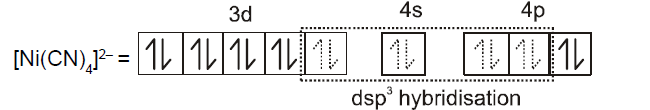•   Question 57

Given are cyclohexanol (I), acetic acid (II), 2, 4, 6-trinitrophenol (III) and phenol (IV). In these the order of decreasing acidic character will be:

A : III > II > IV> I
B : II > III > I > IV
C : II > III > IV > I
D : III > IV > II > I

•
.

Solution :
Higher the tendency to give a proton, higher is the acidic character, and tendency to lose a proton depends upon the stability of intermediate, i.e., carbanion formed.

•   Question 58

If pH of a saturated solution of Ba(OH)2 is 12, the value of its Ksp is:

A : 4.00 * 10 -6 M3
B : 4.00 * 10 -7 M3
C : 5.00 * 10 -6 M3
D : 5.00 * 10 -7 M3

•
.

Solution :
At 25 C pH + pOH = 14
pOH = 2
[OH] = 10�2 M Now Let solulity of Ba(OH)2 be S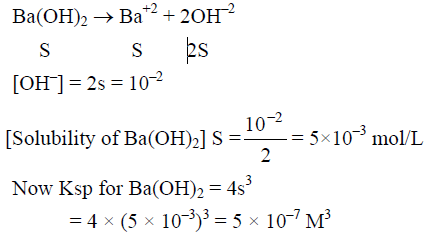•   Question 59

The reaction of toluene with Cl2 in presence of FeCl3 gives 'X' and reaction in presence of light gives 'Y'. Thus, 'X' and 'Y' are:

A : X = Benzal chloride, Y = o-chlorotoluene
B : X =m-chlorotoluene, Y = p-chlorotoluene
C : X = o-and p-chlorotoluene, Y = Trichloromethyl benzene
D : X = Benzyl chloride, Y = m-chlorotoluene

•
.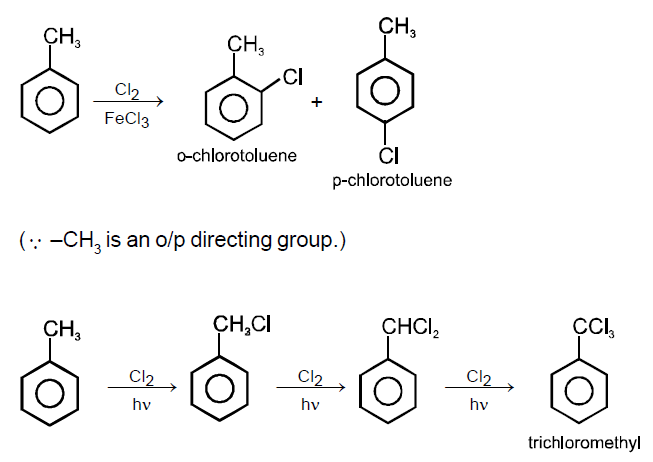•   Question 60

Which one of the following compounds has the most acidic nature?

A :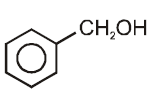B :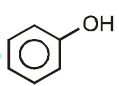C :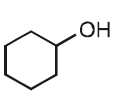D :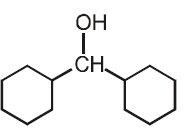•
.

Solution :
Presence of electron withdrawing substituent increases the aciditywhile electron relasing substituent, when present, decreases the acidity. Phenyl is an electron withdrawing substituent while ?CH3 is an electron releasing substituent. Moreover, phenoxide ion is more resonance stabilised as compared to benzyloxide ion, thus releases proton more easily. That?s why is a strong acid among the given.

•   Question 61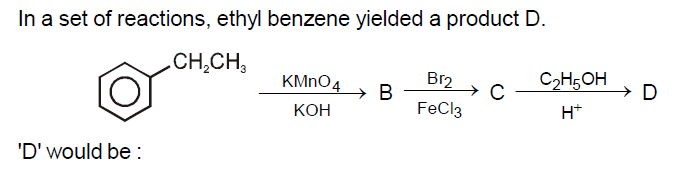A :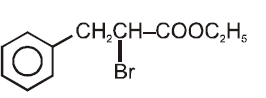B :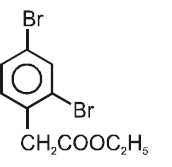C :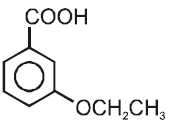D :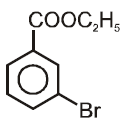•
.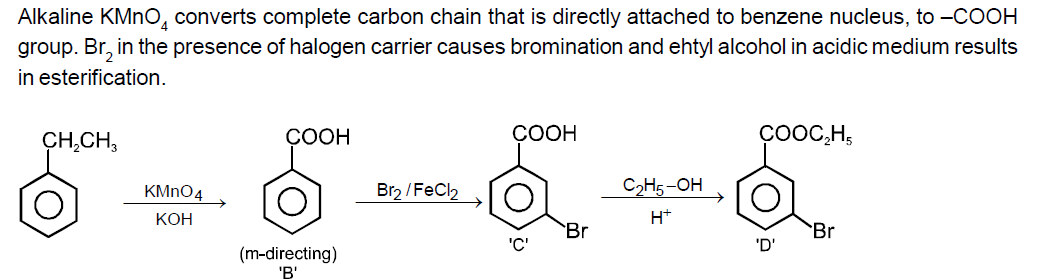•   Question 62

What is [H+] inmol/L of a solution that is 0.20M in CH3COONa and 0.10 M in CH3COOH ? (Ka for CH3COOH = 1.8 * 10-5)

A : 3.5 * 10 -4
B : 1.1 * 10 -5
C : 1.8 * 10 -4
D : 9.0 * 10 -6

•
.

Solution :
For acidic buffer solution
[H+] = Ka[CH3COOH] / CH3COO-]

•   Question 63

For an endothermic reaction, energy of activation is Ea and enthalpy of reaction is ΔH (both of these in kJ/mol). Minimum value of Ea will be:

A : less than ΔH
B : equal to ΔH
C : more than ΔH
D : equal to 0

•
.

Solution :
In endothermic reactions, energy of reactants is less than that of the products. Potential energy diagram for endothermic reactions is,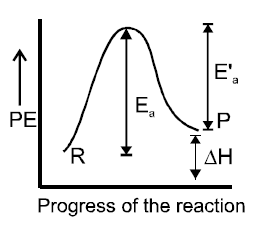•   Question 64

The correct order of increasing reactivity of C ? X bond towards nucleophile in the following compounds is: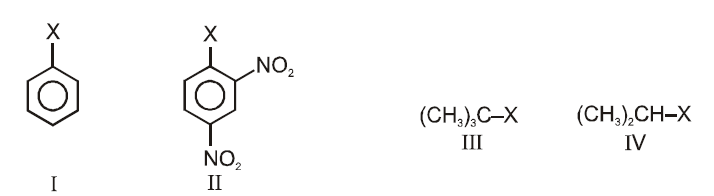A : I < II < IV < III
B : II < III < I < IV
C : IV < III < I < II
D : III < II < I < IV

•
.

Solution :
Alkyl halides are more reactive twoward nucleophilic substitution. Reactivity depends upon the stability of carbocation intermedicate formed.

•   Question 65

For the reduction of silver ions with copper metal, the standard cell potential was found to be + 0.46 V at 25°C. The value of standard Gibbs energy ΔG° will be (F = 96500 C mol-?1)

A : -89.0 kJ
B : -89.0 J
C : -44.5 kJ
D : -98.0 kJ

•
.

Solution :
2Ag+ + Cu → 2Ag + Cu2+
n=2
ΔG = -nFEcell
ΔG = -2 * 96500 * 0.46 Joul
ΔG = -88.78kJ

•   Question 66

In which of the following equilibrium Kc and Kp are not equal?

A :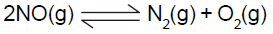B :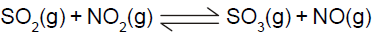C :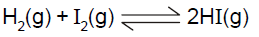D :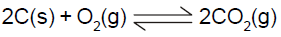•
.

Solution :
Kp = Kc(RT)Δn

•   Question 67

Which of the following ions will exhibit colour in aqueous solutions ?

A : La3+ (Z = 57)
B : Ti3+ (Z = 22)
C : Lu3+ (Z = 71)
D : Sc3+ (Z = 21)

•
.

Solution :
Ti3+ (Z = 22) Ions which have unpaired electrons exhibit colour in solution. Ti3+ has an outer electronic configuration of 4s0 3d1, i.e., 1 unpaired electron. Thus its solution will be coloured. Sc3+ → d0 In case of La3+, 4f0 configuration is present and in Lu3+, 4?f14 is present. So, there is no possibility of ?f-f? transition, hence these ions do not appear coloured.

•   Question 68

Aniline in a set of the following reactions yielded a coloured product 'Y'.

A :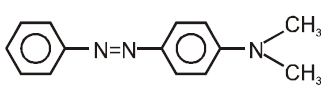B :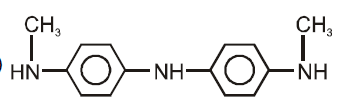C :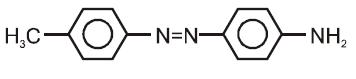D :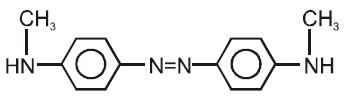•
.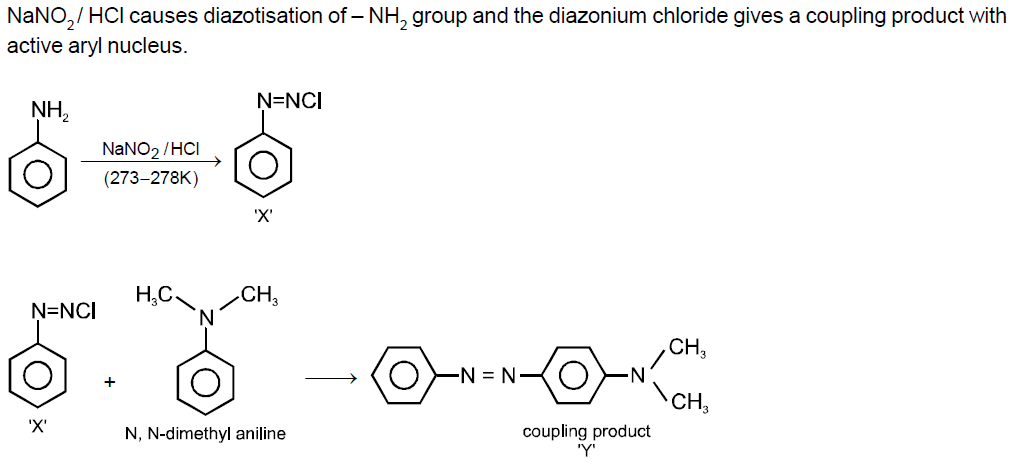•   Question 69

Acetamide is treated with the following reagents separately.Which one of these would yieldmethyl amine ?

A : NaOH-Br2
B : Sodalime
C : Hot conc. H2SO4
D : PCl5

•
.

Solution :
The reagent which can convert -CONH2 group into -NH2 group is used for this reaction. Among the given reagents only NaOH/Br2 converts -CONH3 group to -NH2 group, thus it is used for converting acetamide to methyl amine. This reaction is called Hoffmann bromamide reaction.

•   Question 70

An aqueous solution is 1.00 molal in KI. Which change will cause the vapour pressure of the solution to increase?

C : Addition of 1.00 molal KI

•
.

Solution :
Vapour pressure depends upon the surface area of the solution. Larger the surface area, higher is the vapour pressure. Addition of solute decreases the vapour pressure as some dites of the surface are occupied by solute particles, resulting in decreased surface area. However, addition of solvent(dilution),increases the surface area of the liquid surface, thus results in increased vapour pressure. Hence, addition of water to the aqueous solution of (1 molal) KI, results in increased vapour p

•   Question 71

A solution of sucrose (molar mass = 342 gmol?-1) has been prepared by dissolving 68.5 g of sucrose in 1000 g of water. The freezing point of the solution obtained will be: (Kf for water = 1.86 K kg mol?-1)

A : -0.372°C
B : -0.520°C
C : + 0.372°C
D : -0.570°C

•
.

Solution :
Depression in freezing point
ΔTf = kf * m
where m = molality = (WB * 1000)/MBWA

•   Question 72

Which of the following alkaline earth metal sulphates has hydration enthalpy higher than the lattice enthalpy?

A : CaSO4
B : BeSO4
C : BaSO4
D : SrSO4

•
.

Solution :
Hydration energy varies inversely with size and in sulphates of alkaline earth metals lattice energy remains almost constant. The order of size of alkaline earth metals is
Be2+ < Ca2+ < Sr2+ < Ba2+
Thus, the order of hydration energy is Be2+ > Ca2+ > Sr2+ > Ba2+ Hence, BeSO2 has the hydration enthalpy higher than the lattice enthalpy.

•   Question 73

Which of the following ions has electronic configuration [Ar]3d6 ?

A : Ni3+
B : Mn3+
C : Fe3+
D : Co3+

•
.

Solution :
Write the electronic configurations of given ions and find the correct answer.

•   Question 74

An increase in equivalent conductance of a strong electrolyte with dilution is mainly due to:

A : increase in ionic mobility of ions.
B : 100% ionisation of electrolyte at normal dilution.
C : increase in both i.e. number of ions and ionic mobility of ions.
D : increase in number of ions.

•
.

Solution :
λeq = k * V
=k*1000/normality
On dilution, the number of current carrying particles per cm3 decreases but the volume of solution increases. Consequently, the ionic mobility increases, which in turn increases the equivalent conductance of strong electrolyte.

•   Question 75

Crystal filed stabilization energy for high spin d4 octahedral complex is

A : -1.8 δ0
B : -1.6 δ0 + P
C : -1.2 δ0
D : -0.6 δ0

•
.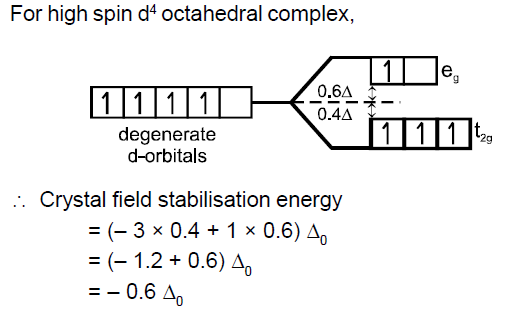•   Question 76

Oxidation states of P in H4P2O5, H4P2O6, H4P2O7 are respectively

A : +3, +5, +4
B : +5, +3, +4
C : +5, +4, +3
D : +3, +4, +5

•
.

Solution :
Oxidation state of H is + 1 and that of O is -2.
Let the oxidation state of P in the given compounds is x.
In H4P2O5, (+1) * 4 + 2 * x + (? 2) * 5 = 0
4 + 2x - 10 = 0
2x = 6
x = + 3
In H4P2O6, (+1) * 4 + 2 * x + (? 2) * 6 = 0
4 + 2x - 12 = 0

•   Question 77

Which of the following statements about primary amines is 'False'?

A : Alkyl amines are stronger bases than aryl amines
B : Alkyl amines react with nitrous acid to produce alcohols
C : Aryl amines react with nitrous acid to produce phenols
D : Alkyl amines are stronger bases than ammonia

•
.

•   Question 78

The correct order of increasing bond angles in the following species are :

A : Cl2O < ClO2 < ClO2- ?
B : ClO2 < Cl2O < ClO2- ?
C : Cl2O < ClO2- < ClO2
D : ClO2- < Cl2O < ClO2

•
.

Solution :
As the number of lone pairs of electrons increases, bond angle decreases due to repulsion between 1p - 1p. Moreover, as the electronegativity of central atom decreases, bond angle decreases. Hence, the order of bond angle is ClO2- < Cl2O < ClO2
(Cl is less electronegative as compared to O.)

•   Question 79

Among the given compounds, the most susceptible to nucleophilic attack at the carbonyl group is:

A : CH3COOCH3
B : CH3CONH2
C : CH3COOCOCH3
D : CH3COCl

•
.

Solution :
Lesser the electron density of acyl carbon atom, more will be the susceptiblity of nucleophile to attack it.
The Cl atom has strong -I effect because of the weak p-bond between the small sized C-atom and large sized Cl atom. Thus in CH3COCl, acyl carbon has least electron density and hence, more suceptible for nucleophilic attack.

•   Question 80

25.3 g of sodium carbonate, Na2CO3 is dissolved in enough water to make 250 mL of solution. If sodium carbonate dissociates completely, molar concentration of sodium ion, Na+ and carbonate ions, CO32- are respectively (Molar mass of Na2CO3 = 106 gmol?-1)

A : 0.955 M and 1.910 M
B : 1.910 M and 0.955 M
C : 1.90 M and 1.910 M
D : 0.477 M and 0.477 M

•
.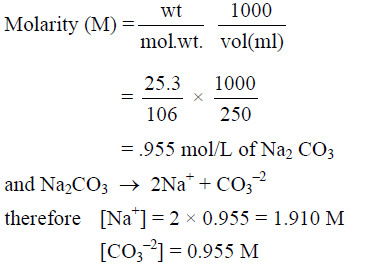•   Question 81

In a buffer solution containing equal concentration of B?-and HB, the Kb for B-? is 10?-10. The pH of buffer solution is:

A : 10
B : 7
C : 6
D : 4

•
.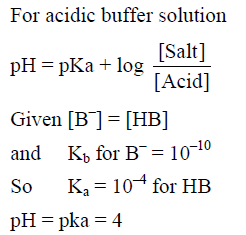•   Question 82

The existance of two different coloured complexes with the composition of [Co(NH3)4Cl2]+ is due to :

B : geometrical isomerism
C : coordination isomerism
D : ionization isomerism

•
.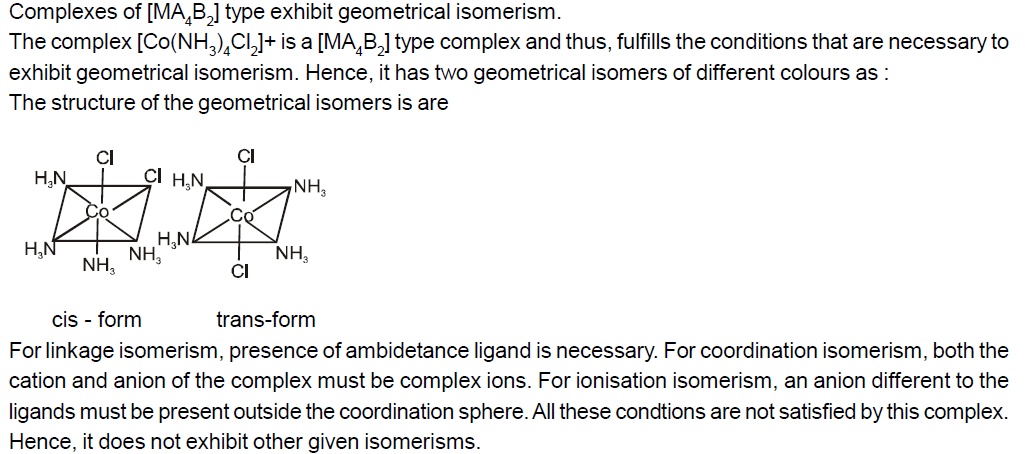•   Question 83

Property of the alkaline earth metals that increases with their atomic number:

A : Solubility of their hydroxides in water
B : Solubility of their sulphates in water
C : Ionization energy
D : Electronegativity

•
.

•   Question 84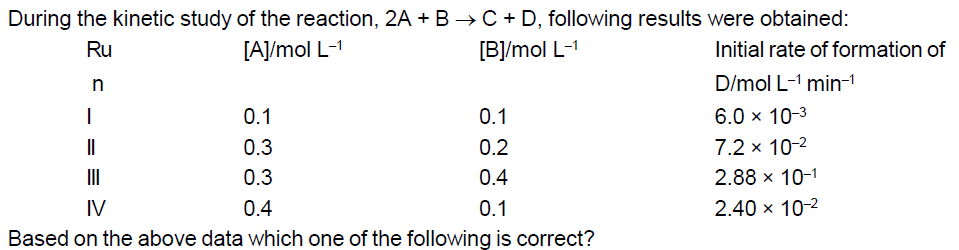A : rate = k[A]2[B]
B : rate = k[A][B]
C : rate = k[A]2[B]2
D : rate = k[A][B]2

•
.

Solution :
For order of A :
By run I & IV
[B] remain same but
[A] increases 4 times and rate of reaction also becomes 4 times
so order w.r.t. A is 1
for order of B
By Run III & III
[A] remains same but
[B] becomes 2 times and rate of reaction becomes 4 times
so order w.r.t. B is 2
so rate = K[A][B]2

•   Question 85

Which of the following pairs has the same size ?

A : Fe2+, Ni2+
B : Zr4+, Ti4+
C : Zr4+, Hf4+
D : Zn4+, Hf4+

•
.

Solution :
In general, the atomic and ionic radii increases onmoving down a group. But the elements of second series (eg, Zr, Nb,Mo etc.) have the almost same radii as the elements of third transition serries (eg,Hf, Ta,W etc). This is because of lanthanide contraction ie, imperfect sheilding of one 4f - electron by another.

•   Question 86

The correct order of the decreasing ionic radii among the following is electronic species are:

A : Ca2+ > K+ > S?2-> Cl?-
B : Cl?- > S2- > Ca2+ > K+
C : S2- > Cl-? > K+ > Ca2+
D : K+ > Ca2+ > Cl?- > S22-

•
.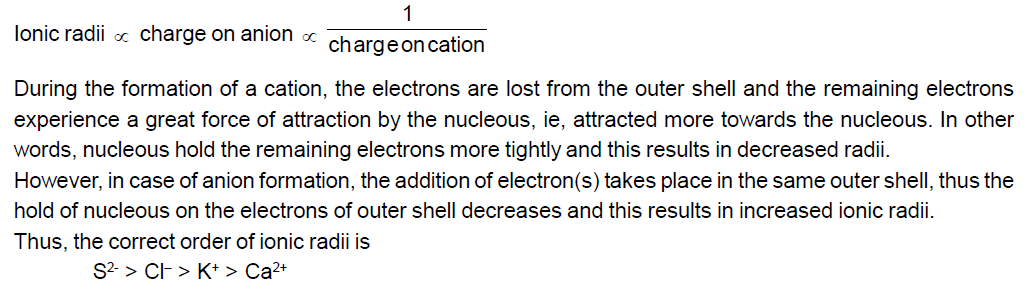•   Question 87

In which one of the following species the central atom has the type of hybridization which is not the same as that present in the other three?

A : SF4
B : I3-
C : SbCl52-
D : PCl5

•
.

Solution :
Since, only SbCl52- has different number of hybrid orbitals (ie, 6) from the other given species, its hybrisation is different from the others, ie, sp3d2. (The hybridsation of other species is sp3d).

•   Question 88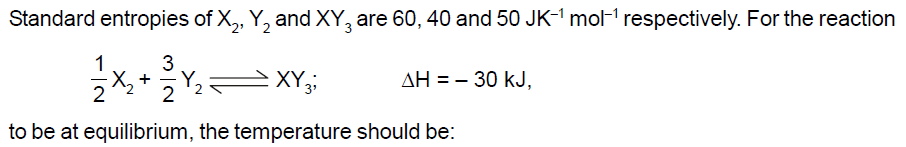A : 750 K
B : 1000 K
C : 1250 K
D : 500 K

•
.

Solution :
ΔS = ∑SP - ∑SR
ΔS = 50 - (1/2 * 60 + 3/2*40)
ΔS = -40
ΔG = ΔH -TΔS
at equilibrium ΔG = 0
T= ΔH / ΔS
T=750 K

•   Question 89

Which of the following represents the correct order of increasing electron gain enthalpywith negative sign for the elements O, S, F and Cl ?

A : Cl < F < O < S
B : O < S < F < Cl
C : F < S < O < Cl
D : S < O < Cl < F

•
.

Solution :
the electron gain enthalpy of Cl and F is higher as compared to O and S. Cl and F > O and S
Between Cl and F, Cl has higher electron gain enthalpy as in F, the incoming electron experiences a greater force of repulsion because of small size of F atom. Similar is true in case of O and S ie, the electron gain enthalpy of S is higher as compared to O due to its small size. Thus, the correct order of electron gain enthalpy of given elements is O < S < F< Cl

•   Question 90

Which one of the following compounds is a peroxide ?

A : KO2
B : BaO2
C : MnO2
D : NO2

•
.

•   Question 91

Which one is most reactive towards electrophilic reagent ?

A :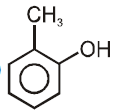B :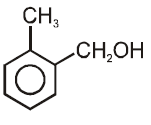C :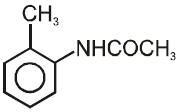D :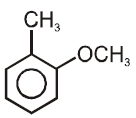•
.

Solution :
Electron withdrawing substituent deactivates the benzene nucleous towards electrophilic substitution while electron releasing substituent activates the ring towards electrophilic substitution. Among the given, - OH has the higher electron donating tendency and thus, activates the ringmore towards electrophilic substitution. Hence, is more reactive towards electrophilic reagent.

•   Question 92

Which one of the following is employed as a Tranquilizer drug?

A : Promethazine
B : Valium
C : Naproxen
D : Mifepristone.

•
.

Solution :
Tranquilizer are the chemicals that reduce anxiety and mental tension. Thus, they are sometimes called psychotherapteutic drugs. Equanil, valium and serotonin are some commonly used transquilizers.

•   Question 93

In the following the most stable conformation of n-butane is : (

A :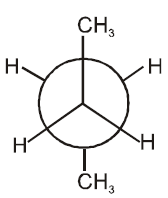B :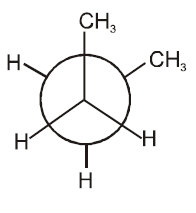C :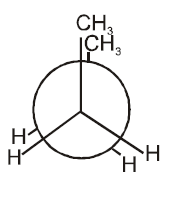D :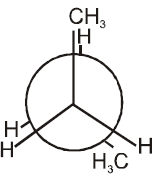•
.

Solution :
The conformation in which the heavier groups are present atmaximum possible distances, so that the forces of repulsion get weak, is more stable. Among the given conformation of n-butane, the conformation ismost stable as in it the bulkier group (ie, CH3 group) are present at maximum possible distance.

•   Question 94

Which of the following reactions will not result in the formation of carbon-carbon bonds?

A : Reimer-Tieman reaction
B : Cannizaro reaction
C : Wurtz reaction
D : Friedel-Crafts acylation

•
.

Solution :
2HCHO → CH3OH + HCOONa
(No new C - C bond is formed in this reaction.)

•   Question 95

Which of the following structures represents Neoprene polymer ?

A :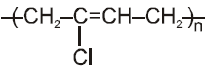B :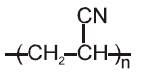C :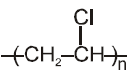D :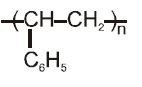•
.

Solution :
Neoprene (synthetic rubber) is a polymer of chloroprene (ie, 2-chloro -1, 3 - butadiene).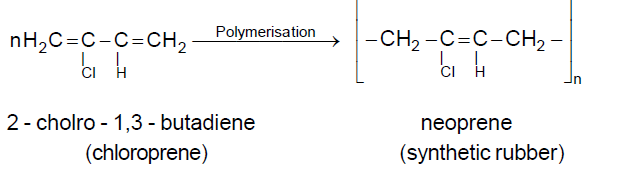•   Question 96

Which one is most reactive towards SN1 reaction?

A : C6H5CH(C6H5)Br
B : C6H5CH(CH3)Br
C : C6H5C(CH3)(C6H5)Br
D : C6H5CH2Br

•
.

Solution :
SN1 reaction involves the formation of carbocation intermediate.More the stability of carbocation, more is the reactivity of alkyl/aryl haldies towards SN1 reaction.

•   Question 97

AB crystallizes in a body centred cubic lattice with edge length 'a' equal to 387 pm. The distance between two oppositely charged ions in the lattice is:

A : 335pm
B : 250pm
C : 200pm
D : 300pm

•
.

Solution :
For body centred cubic (bcc) lattice, distance between two oppositely charged ions,
d= √3a/2 = √*387/2pm
=334.15pm

•   Question 98

The number of atoms in 0.1 mol of a triatomic gas is: (NA = 6.02 * 1023 mol-?1)

A : 6.026 * 1022
B : 1.806 * 1023
C : 3.600 * 1023
D : 1.800 * 1022

•
.

Solution :
Number of atoms = number of moles * NA * atomicity
=0.1 * 6.02 * 10 23 * 3 =1.806 * 10 23

•   Question 99

Which one of the following molecular hydrides acts as a Lewis acid ?

A : NH3
B : H2O
C : B2H6
D : CH4

•
.

Solution :
Electron deficient molecules behave as Lewis acid. Among the given molecules, only dibroane is electron deficient, i.e. does not have complete octet. Thus, it acts as a Lewis acid.

•   Question 100

The tendency of BF3, BCl3 and BBr3 to behave as Lewis acid decreases in the sequence :

A : BCl3 > BF3 > BBr3
B : BBr3 > BCl3 > BF3
C : BBr3 > BF3 > BCl3
D : BF3 > BCl3 > BBr3

•
.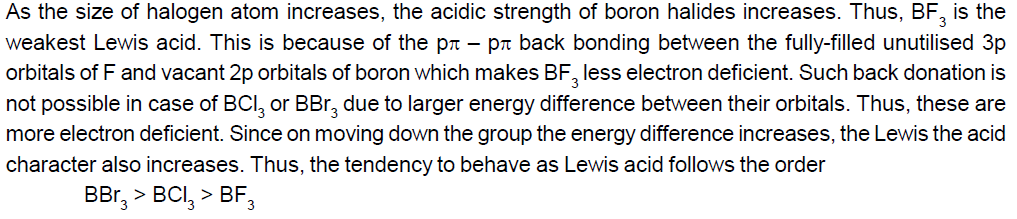•   Question 101

Apomictic embryos in citrus arise form :

A : Maternal sporophytic tissue in ovule
B : Antipodal cells
C : Diploid egg
D : Synergids

•
.

•   Question 102

If due to some injury the chordae tendinae of the tricuspid valve of the human heart is partially non-functional, what will be the immediate effect

A : The pacemaker will stop working
B : The blood will tend to flow into the leftatrium
C : The flow of blood into the pulmonary arterywill be reduced
D : The flow of blood into the aorta will be slowed down

•
.

•   Question 103

The nerve centres which control the body temperature and the urge for eating are contained in

A : Pons
B : Cerebellum
C : Thalamus
D : Hypothalamus

•
.

•   Question 104

The plasmamembrane consistsmainly of

A : Proteins embedded in a phospholipid bilayer
B : Proteins embedded in a polymer of glucosemolecules
C : Proteins embedded in a carbohydrate bilayer
D : Phospholipids embedded in protein bilayer

•
.

•   Question 105

In unilocular ovary with a single ovule the placentation is

A : Basal
B : Free Central
C : Axile
D : Marginal

•
.

•   Question 106

The genetically-modified (GM) brinjal in India has been developed for

A : Enhancing shelf life
B : Enhanicing mineral content
C : Drought-resistance
D : Insect-resistance

•
.

•   Question 107

Ringworm is humans is caused by

A : Fungi
B : Nematodes
C : Viruses
D : Bacteria

•
.

•   Question 108

Which one of the following pair is incorrectlymatched

A : Somatostatin - Delta cells (Source)
B : Corpusluteum - Relaxin (secretion)
C : Insulin - Diabetes mellitus (disease)
D : Glucagon - Beta cells (source)

•
.

•   Question 109

Widal test is used for the diagnosis of

A : Pneumonia
B : Tuberculosis
C : Typhoid
D : Malaria

•
.

•   Question 110

Which one of the following is an example of ex-situ conseryation

A : Seed bank
B : Sacred groves
C : National park
D : Wildlife sanctuary

•
.

•   Question 111

Whcih one of the following symbols and its representation used in human pedigree analysis is correct

A :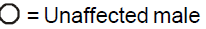B :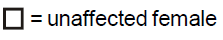C :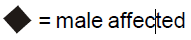D :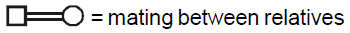•
.

•   Question 112

The permissible use of the technique amniocentesis is for

A : Artificial insemination
B : Transjfer of embryo into the uterus of a surrogatemother
C : Detecting any genetic abnormality
D : Detecting sex of the unborn foetus

•
.

•   Question 113

Some hyperthermophilic organisms that grow in highly acidic (pH2) habitats belong to the two groups

A : Cyanobacteria and diatoms
B : Protists and mosses
C : Liverworts and yeasts
D : Eubacteria and archaea

•
.

•   Question 114

Which one of the following statements in regard to the excretion by the human kidneys is correct

A : Distal convoluted tubule is incapable of reabsorbing HCO2- ?
B : Nearly 99 per cent of the glomerular filtrate is reabsorbed by the renal tubules
C : Ascending limb of loop of Henle is impermeable to electrolytes
D : Descending limb of loop of Henle is impermeable to water

•
.

•   Question 115

Which one of the following statements in regard to the excretion by the human kidneys is correct

A : Distal convoluted tubule is incapable of reabsorbing HCO2- ?
B : Nearly 99 per cent of the glomerular filtrate is reabsorbed by the renal tubules
C : Ascending limb of loop of Henle is impermeable to electrolytes
D : Descending limb of loop of Henle is impermeable to water

•
.

•   Question 116

Which one of the following is not lateral meristem

A : Interfascicular cambium
B : Phellogen
C : Intercalarymeristem
D : Intrafascicular cambium

•
.

•   Question 117

Single-celled eukaryotes included in

A : Fungi
B : Archaea
C : Monera
D : Protista

•
.

•   Question 118

C4 plants are more efficient in photosynthesis than C3 plants due to

A : Presence of larger number of chloroplasts in the leaf cells
B : Presence of thin cuticle
C : Lower rate fo photorespiration
D : Higher leaf area

•
.

•   Question 119

Which one of the following is used as vector for cloning genes into higher organisms

A : Salmonell typhimurium
B : Rhizopus nigricans
C : Retrovirus
D : Baculovirus

•
.

•   Question 120

Which one of the following is not a micronutrient

A : Magnesium
B : Zinc
C : Boron
D : Molybdenum

•
.

•   Question 121

One example of animal having a single opening to the ouside that serves both as mouth as well as anus is

A : Asterias
B : Ascidia
C : Fasciola
D : Octopus

•
.

•   Question 122

Which one of the following structure between two adjacent cells is an effective transport pathway

A : Plastoquinones
B : Endoplasmic reticulum
C : Plasmalemma
D : Plasmodesmata

•
.

•   Question 123

Which one of the following does not follow the central dogma of molecular biology

A : Mucor
B : Chlamydomonas
C : HIV
D : Pea

•
.

•   Question 124

Study the four statements (a-d) given below and select the two correct ones out of them
(a)Alion eating a deer and a sparrow feeding on grain are ecologically similar in being consumers
(b) Predator star fish pisaster helps in maintaining species diverstiy of some invertebrates
(c) Predators ultimately lead to the extinction of pery species
(d) Production of chemicals such as nicotine, strychnine by the plants are metabolic disorders
The two correct statements are

A : (c) and (d)
B : (a) and (d)
C : (a) and (b)
D : (b) and (c)

•
.

•   Question 125

Toxic agents present in food which interfere with thyroxine synthesis lead ot the development of

A : cretinism
B : simple goitre
C : thyrotoxicosis
D : toxic goitre

•
.